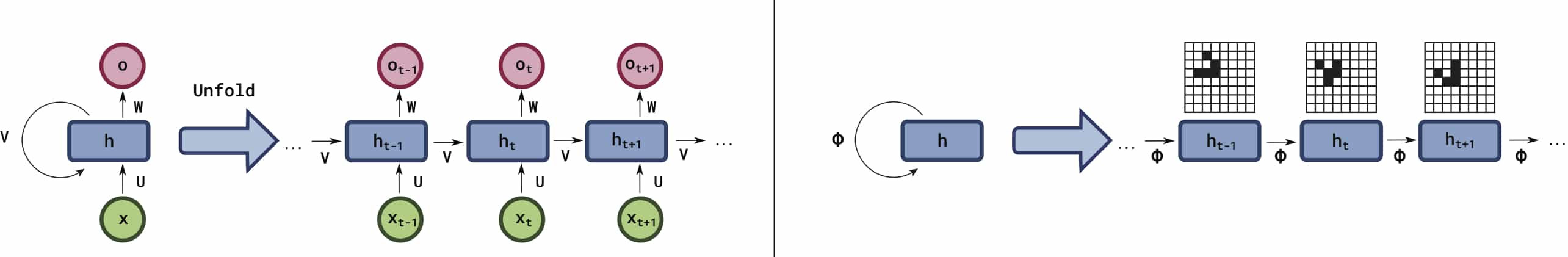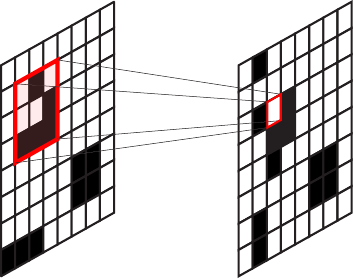# Cellular automata as convolutional neural networks

tags
Cellular automata, Convolutional neural networks

## Motivation

Cellular automata are computational models based on several principles such as translation-invariance and parallelism of computations. These principles also motivated the creation of Convolutional neural networks — used initially for images and text —, making this model well-suited to reason about cellular automata.

There is indeed a deep connection between the two models, making it seem like there are two expressions of the same idea: spatially organized information can be processed locally in parallel.

Here is an example with the Game of Life:Figure 1: Any cell update in the Game of Life could be expressed with a neural network, making the global update a series of convolutions + non-linearities

## Relevant literature nnca

Cellular automata can easily be represented as Convolutional Neural Networks (CNNs). This relation has already been investigated in (Gilpin 2018) or (Mordvintsev et al. 2020) for instance. This idea has advantages and drawbacks:

• Computational cost of using floating points operations is higher than discrete operations.
• Discrete operations can lead to exponential growth of the number of possible configurations and update rules in a CA. This is why rules aren’t represented as bijective tables matching neighborhoods to states anymore in (Mordvintsev et al. 2020).
• The use of floating point operations is mainly there to be able to compute gradients for gradient based optimization. This is against a non-supervised philosophy I am trying to work on. Training cellular automata is basically re-implementing a “recurrent convolutional neural network”: this might lead to interesting models but isn’t exploiting all the potential of those computational models
• Ultimately computations in a computer are discrete and Mordvintsev et al. eventually use quantization to discretize the CA, resulting in an approximation of a discrete automaton.

## Graph networks

CNNs could be considered a particular architecture of neural network especially designed for regular grid-like graphs.

However, a CA can have arbitrary structures as long as the rule is defined to deal with this type of structures. This extends the concept of CA-CNN to graph neural networks.

## Turing-completeness

Turing-completeness of convolutional neural networks can be directly inferred from this close relation with cellular automata. The condition is to consider a recurrent-convolutional network where the output is fed back to the network at each step. A simple ECA rule such as rule 110 is easily implemented in this type of convolutional neural network, hence Turing-completeness.

## Bibliography

1. . . "Cellular Automata as Convolutional Neural Networks". Arxiv:1809.02942 [cond-mat, Physics:nlin, Physics:physics]. http://arxiv.org/abs/1809.02942. See notes
2. . . "Growing Neural Cellular Automata". Distill 5 (2):e23. DOI. See notes